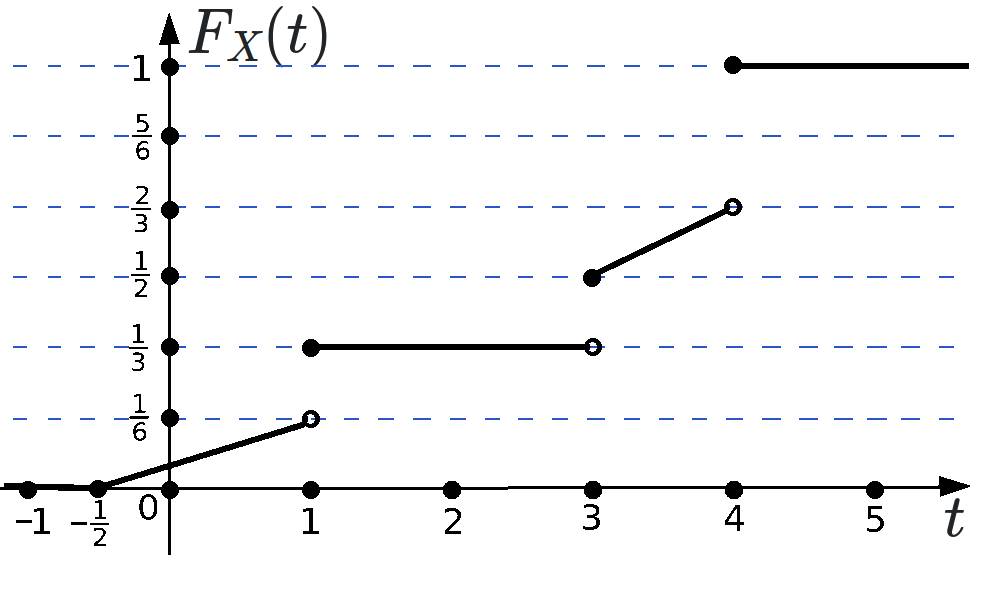# MTH4120: Midterm 1 Practice 3

Problem 1.

It is known that $$(\Omega,\mathcal F,\mathbb P)$$ is a probability space. It is known that and $$M$$ and $$N$$ are two events on this probability space. Which of the following objects is a properly defined real number?

• (A) $$M+\mathbb P(N)$$
• (B) $$\mathbb P(M\cap N)$$
• (C) $$\mathbb P\Big(\left\{M=10\right\}\cup\left\{N=11\right\}\Big)$$
• (D) $$\mathbb P\Big( M \cup\left\{N=11\right\}\Big)$$
• (E) $$\mathbb E[M]+\mathbb P(N)$$

Problem 2.

The sample space $$\Omega$$ is given by $$\Omega=\Big\{ y, \{x\}, \{x,y\}, \{y,\{x,y\}\} \Big\}$$. Which of the following objects is an outcome but not an event?

• (A) $$\mathbb P:\Omega\to [0,1]$$
• (B) $$\mathbb P:\mathcal F\to [0,1]$$
• (C) $$\{x,y\}$$
• (D) $$\{\{x\}, y\}$$
• (E) $$\{y,\{x,y\}\}$$

Problem 3. The events $$A$$ and $$B$$ on probability space $$(\Omega, \mathcal F,\mathbb P)$$ satisfy $$\mathbb P\left(A^C\cap B\right)=0.31$$, $$\mathbb P\left(A\cup B\right)=0.79$$, and $$\mathbb P\left(A\cap B\right)=0.25$$. Evaluate $$100\cdot \mathbb P\left(A\right)$$.

Problem 4. A group of friends plans to visit only these four groups of animals at the zoo: aligators, bees, camels, and dolphins. They are deciding on the order in which the animals should be visited. One of the following schedules will be chosen at random:
• 1. Visit the aligators first, then bees, then camels, and finally dolphins.
• 2. Visit the dolphins, then aligators, then camels, then bees.
• 3. Visit the camels, then dolphins, then aligators, then bees.
• 4. Visit the bees, then aligators, then dolphins, then camels.
• 5. Visit the camels, then bees, then aligators, then dolphins.
Each of the above five plans has equal chance of being selected. Construct the probability space $$(\Omega,\mathcal F, \mathbb P)$$ that corresponds to the described random experiment. Determine the set that corresponds to the event that the dolphins are visited before the aligators.

Problem 5. The random variables $$X$$ and $$Y$$ are independent. The codomain of both random variables $$X$$ and $$Y$$ is the set $$\{10,11,12,\dots, 110\}$$. It is known that $$\mathbb P(X=10)= \frac{ 3 }{11}$$, $$\mathbb P(Y=11)= \frac{ 2 }{11}$$, and $$\mathbb P\left(\left.Y >11\right|X >12\right)=\frac{15}{22}$$. What is $$242\cdot \mathbb P(X+Y=20)$$?

Problem 6. Evaluate $$18\cdot \mathbb P(X=0)+90\cdot \mathbb P\left(X\in(2,4]\right)-18\cdot \mathbb P\left(X\in(3,5]\right)$$, where $$X$$ is a random variable whose cumulative distribution function $$F_X(t)$$ has the graph given in the picture below.Problem 7. The probability density function $$f_X$$ of the continuous random variable $$X$$ satisfies $f_X(t)=\left\{\begin{array}{ll} 0, &\mbox{if } t\leq -14;\\ \frac{t+14}{196},&\mbox{if }t\in\left(-14,0\right];\\ \frac{14-t}{196},&\mbox{if }t\in\left(0,14\right);\\ 0,&\mbox{if }t\geq 14. \end{array}\right.$ Evaluate $$196\cdot \mathbb P\left(X\in \left(-5,9\right)\right)$$.

Problem 8. Prove that there exists a probability space $$(\Omega, \mathcal F, \mathbb P)$$ and two random variables $$X$$ and $$Y$$ on this probability space such that $\mathbb E\left[X\right]=4,\quad \mathbb E\left[Y\right]=7,\quad \mbox{ and }\quad \mathbb E\left[XY\right]=2.$